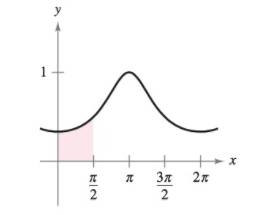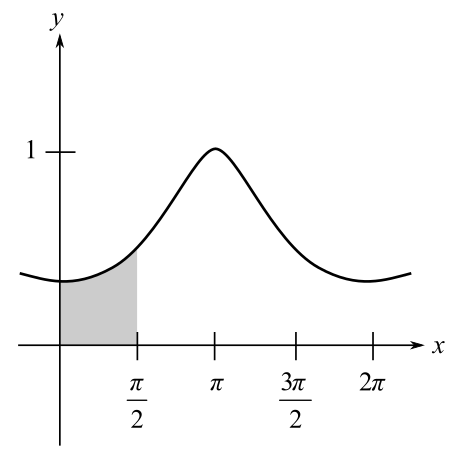Chapter 8, Problem 5PS

Chapter
Section
Textbook Problem

Area Use the substitution u = tan x 2 vto find the area of the shaded region under the graph of y = 1 2 + cos x for 0 ≤ x ≤ π / 2 (see figure).To determine

To calculate: The area of the shaded region in the given graph by using substitution of u=tanx2.Explanation

Given:

The function for the graph is y=12+cosx for [0,π2].

The given region is as shown below.

Formula used:

The trigonometric formula

cos2A=1tan2A1+tan2A

ddx(tanx)=sec2x

sec2x=tan2x+1

Calculation:

The value is taken as u=tanx2.

Differentiate the above given equation with respect to x.

ddxu=ddx(tanx2)=12sec2x2

Multiplying dx on both sides of the above equation, we get

du=12sec2x2dxdu=1+tan2x22dxdx=21+tan2x2dudx=21+u2du

As the function is y=12+cosx, use the formula

cosx=1tan2x21+tan2x2=1u2<

Still sussing out bartleby?

Check out a sample textbook solution.

See a sample solution

The Solution to Your Study Problems

Bartleby provides explanations to thousands of textbook problems written by our experts, many with advanced degrees!

Get Started

11.If

Mathematical Applications for the Management, Life, and Social Sciences

Write the sum in expanded form. 7. i=1ni10

Single Variable Calculus: Early Transcendentals

True or False: converges.

Study Guide for Stewart's Multivariable Calculus, 8th

For , an upper bound estimate for s − s6 (where s6 is the sixth the series does not converge

Study Guide for Stewart's Single Variable Calculus: Early Transcendentals, 8th